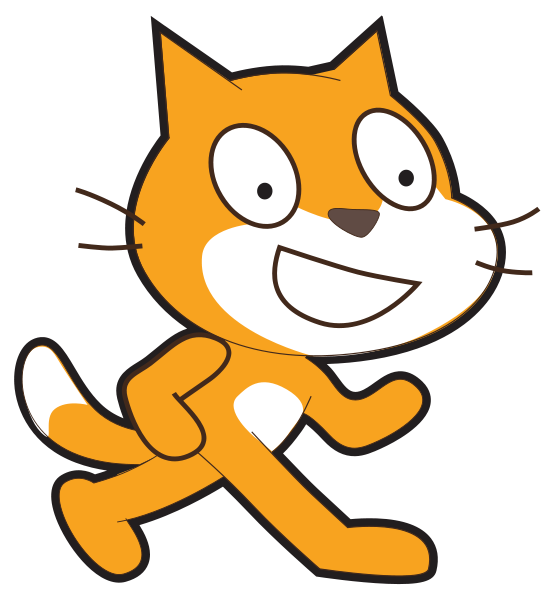# Reflection

Challenge Level: Growing experience

Learning outcomesStudents will be able to:

## Requirement:

Write a program that draws the reflection of the blue shape on the left about the y-axis line. Use two sprites drawing the blue and the red shapes simultaneously.

## Testing examples:

There are no testing examples for this challenge.

## Languages

Scratch#### What it should look like

Click on the green flag to see the expected output of your program.

Recommended blocks for solution 1
when green flag clicked

when green flag clicked
clear

clear

set pen size to (3)

set pen color to [#2ca5e2]

pen down

pen up

set pen size to (3)

set pen color to [#db0705]

pen down

pen up
wait (1) secs

wait (1) secs

wait (1) secs

wait (1) secs

wait (1) secs

wait (1) secs

wait (1) secs

wait (1) secs

wait (1) secs

wait (1) secs

wait (1) secs

wait (1) secs

wait (1) secs

wait (1) secs
go to x: (0) y: (100)

go to x: (-100) y: (100)

go to x: (-100) y: (0)

go to x: (-100) y: (0)

go to x: (-200) y: (0)

go to x: (-200) y: (-100)

go to x: (0) y: (-100)

go to x: (0) y: (100)

go to x: (0) y: (100)

go to x: (100) y: (100)

go to x: (100) y: (0)

go to x: (100) y: (0)

go to x: (200) y: (0)

go to x: (200) y: (-100)

go to x: (0) y: (-100)

go to x: (0) y: (100)
Recommended blocks for solution 2
when green flag clicked

when green flag clicked
wait (1) secs

wait (1) secs

wait (1) secs

wait (1) secs

wait (1) secs

wait (1) secs

wait (1) secs

wait (1) secs

wait (1) secs

wait (1) secs

wait (1) secs

wait (1) secs

wait (1) secs

wait (1) secs
clear

set pen size to (3)

set pen color to [#05d382]

pen down

pen up

clear

set pen size to (3)

set pen color to [#e81818]

pen down

pen up
set [angle v] to 

set [mirror angle v] to ((360) - (angle))
go to x: (0) y: (0)

point in direction (0 v)

move (100) steps

turn ccw (angle) degrees

move (100) steps

turn ccw (angle) degrees

move (100) steps

turn cw (angle) degrees

move (100) steps

turn ccw (angle) degrees

move (100) steps

turn ccw (angle) degrees

move (200) steps

turn ccw (angle) degrees

move (100) steps

go to x: (0) y: (0)

point in direction (0 v)

move (100) steps

turn ccw (mirror angle) degrees

move (100) steps

turn ccw (mirror angle) degrees

move (100) steps

turn cw (mirror angle) degrees

move (100) steps

turn ccw (mirror angle) degrees

move (100) steps

turn ccw (mirror angle) degrees

move (200) steps

turn ccw (mirror angle) degrees

move (100) steps
Hints

Two different solutions to draw the shape and its reflection;

• Solution 1: By drawing lines connecting the vertices (corners) using the “goto” block.

• Solution 2: By moving steps and turning angles (each reflected angle is 180 - original angle).